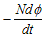# SSC Junior Engineer Exam 20145/2016 Technical Section Answer Key

 Question Answer Electrical resistivity p is High for copper and low for alloy For the circuit shown, the Norton’s equivalent current source at terminals A & B is given by 10∠0 A If the number of turns of a coil is increased, its inductance is increased The unit for permeability is Wb/At´m The internal resistance of a voltage source is 10 Ω and has 10 at its terminals. Find the maximum that can be transferred to the load 2.5 W The voltage across the 1 K Ω resistor of the network shown in the given figure is 2 V Mutual inductance between two coils is 4 H. If current in one coil changes at the rate of 2 A/sec, Then emf induced in the other coil is 8 V
 The e.m.f. indced in a coil of N turns is given byNext›
Please report if you find any errors in the questions.
Keep watching the spaceThanks for reading SSC Junior Engineer Exam 20145/2016 Technical Section Answer Key

←Previous
« Prev Post
Next→
Next Post »